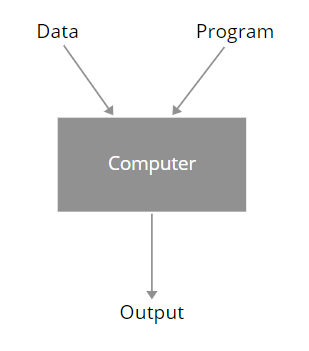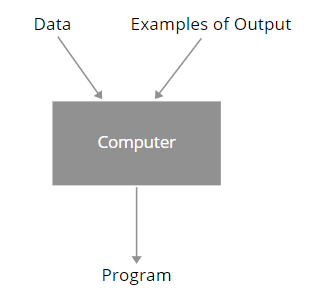Prerequisites to study: None

How does a Machine Learning Solution differ from a Programmatic Solution?

When we have a program or an algorithm to solve a problem, we can represent this system where our computer or processor takes Data and Program as input and returns an output. This is a very general process. For example, suppose we have written a program in C programming language. So when we compile and run the program we give input data and program, as inputs to the computer and the computer returns an output.On the other hand, when we use Machine Learning, we have a system where the computer takes input data and examples of outputs, as inputs and returns a program as output which would solve our problem. So this is how Machine Learning works.What is Machine Learning?

The ability to improve one’s behavior based on experience is known as Learning.

Machine Learning explores algorithms that learn from data, builds models from data and this model can be used for different tasks. For example, the model can be used for prediction, decision making, or solving tasks.

Tom Mitchell’s definition of Machine Learning

A computer program is said to learn from experience E for some class of tasks T and performance measure P if its performance on tasks T as measured by P improves with experience E.

Components of a Machine Learning Algorithm